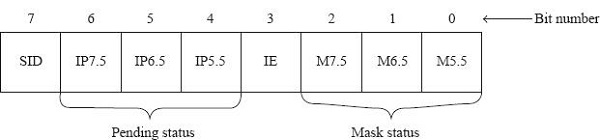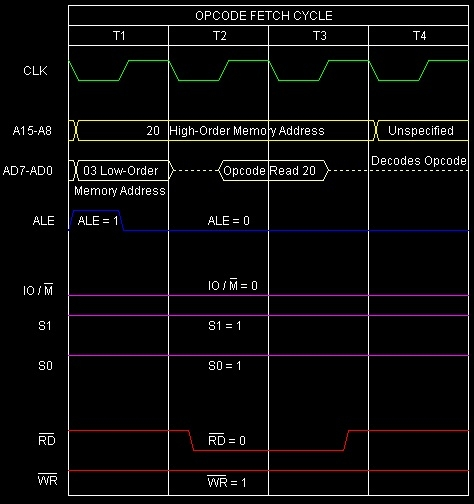# RIM instruction in 8085

Microprocessor8085Microcontroller

In 8085 Instruction set, Read Interrupt Mask. It is a 1-Byte multi-purpose instruction. It is used for the following purposes.

• To check whether RST7.5, RST6.5, and RST5.5 are masked or not;

• To check whether interrupts are enabled or not;

• To check whether RST7.5, RST6.5, or RST5.5 interrupts are pending or not;

• To perform serial input of data.

Mnemonics, Operand
Opcode (in HEX)
Bytes
RIM
20
1

To get the status information about the interrupt system, Read Interrupt Mask instruction provides status information about interrupt system and this instruction can be used for serial input of data. Through this RIM instruction, 8085 can know which interrupt is masked or unmasked, etc. The contents of the Accumulator after the execution of the RIM instruction provide this information.

Thus, it is essential to look into the Accumulator contents after the Read Interrupt Mask instruction is executed. The meaning of the various bits of the Accumulator after Read Interrupt Mask is executed is shown in the following figure –Bit No.
Purpose
Bit 0:
This is mask RST5.5 (M5.5) bit.
• If M5.5 = 1, it means that RST5.5 interrupt has been masked.

• If M5.5 = 0, RST5.5 interrupt is unmasked.

Bit 1:
This is mask RST6.5 (M6.5) bit.
• If M6.5 = 1, it means that RST 6.5 interrupt has been masked.

• If M6.5 = 0, RST 6.5 interrupt is unmasked.

Bit 2:
This is mask RST7.5 (M7.5) bit.
• If M7.5 = 1, it means that RST 7.5 interrupt has been masked.

• If M7.5 = 0, RST7.5 interrupt is unmasked.

Interrupt Enable (IE) status: Bit 3 of the Accumulator provides the status of IE flip-flop after the Read Interrupt Mask instruction is executed.

• If IE = 1, it means that the interrupt system is enabled. This will be the situation if EI instruction is executed sometime prior to the RIM instruction.

• If IE = 0, it means that the interrupt system is disabled. This will be the situation if sometime prior to execution of the RIM instruction, one of the following things have occurred.

• DI instruction was executed

• Intel 8085 has been reset

• Intel 8085 has entered an interrupt service subroutine.

Let us now other status bits in the following table –

Bit No.
Purpose
Bit 4:
This is interrupt pending RST5.5 (IP5.5) bit.
• If IP5.5 = 1, it means that RST5.5 interrupt is pending, waiting to be serviced. This will be the situation when RST5.5 interrupt pin is activated, but RST5.5 is masked, or the interrupt system is disabled.

• If IP5.5 = 0, the RST5.5interrupt is not pending.

Bit 5:
This is interrupt pending RST6.5 (IP6.5) bit.
• If IP6.5 = 1, it means that RST6.5 interrupt is pending, waiting to be serviced.

• If IP6.5 = 0, the RST6.5interrupt is not pending.

Bit 6:
This is interrupt pending RST7.5 (IP7.5) bit.
• If IP7.5 = 1, it means that RST7.5 interrupt is pending, waiting to be serviced.

• If IP7.5 = 0, the RST7.5 interrupt is not pending.

8085 Microprocessor is having one pin labelled Serial Input Data. Which is required for serial data communication. 1-bit of information can be read by the Microprocessor at each single clock pulse. So most significant bit of the Accumulator receives the data present on the SID pin of 8085 when the RIM instruction is executed. In view of this, the meaning of bit 7 of the Accumulator will be as follows, after we execute the RIM instruction.

Bit No.
Purpose
Bit 7:
This is SID bit. After the RIM instruction is executed, the data on the SID pin of 8085 gets

Let us discuss details about the various interrupts of 8085 as summarized in the table that follows.

Pin
Priority
Sensitivity
Condition
TRAP
Highest
0024H
RisingEdge and High Level
Unconditional
RST7.5
Second
003CH
RisingEdge
RST6.5
Third
0034H
High Level
RST5.5
Fourth
002CH
High Level
INTR
Lowest
Supplied by an external device
High Level
EI

Let us consider the following program segment –

Hex Codes
Mnemonic
Comment
2000
3E
MVI A, 19H
A ← 19H =0001 1001, it means SOD=0, SDE=0, R7.5=1, MSE=1, M5.5=1
2001
19

Accumulator value 19H
2002
30
SIM